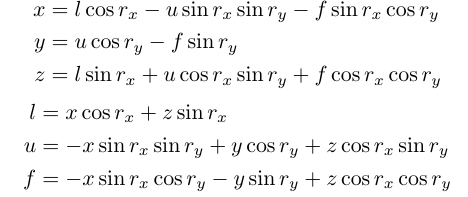# 相对坐标和局部坐标的转换

l: (cos rx,0,sin rx)
u: (-sin rx sin ry, cos ry, cos rx sin ry)
f: (-sin rx cos ry, -sin ry, cos rx cos ry)

x=l cos rx -u sin rx sin ry-f sin rx cos ry
y=u cos ry-f sin ry
z=l sin rx+u cos rx sin ry+f cos rx cos ry

l=x cos rx +z sin rx
u=-x sin rx sin ry+y cos ry+z cos rx sin ry
f=-x sin rx cos ry-y sin ry+z cos rx cos ry(cos rx   -sin rx sin ry    -sin rx cos ry)
(0          cos ry             -sin ry          )
(sin rx    cos rx sin ry     cos rx cos ry)